Learn all Concepts of Linear Equations Class 9 (with VIDEOS). Check - Linear Equations in 2 Variables Class 9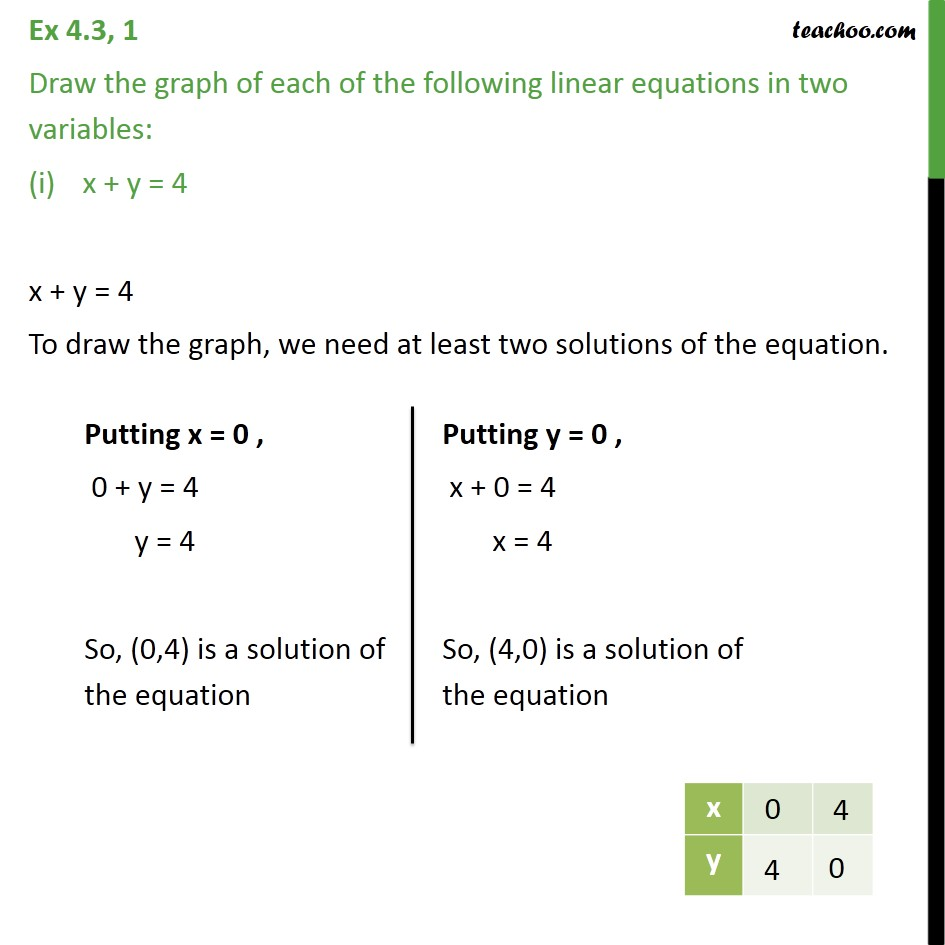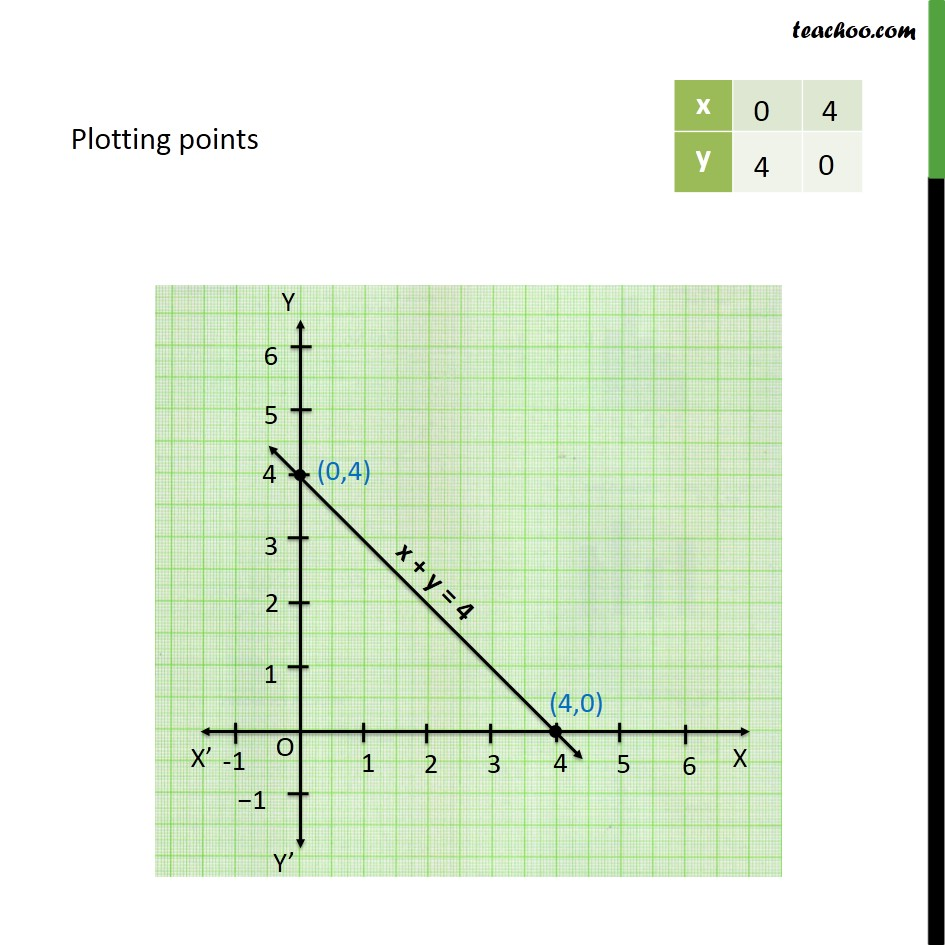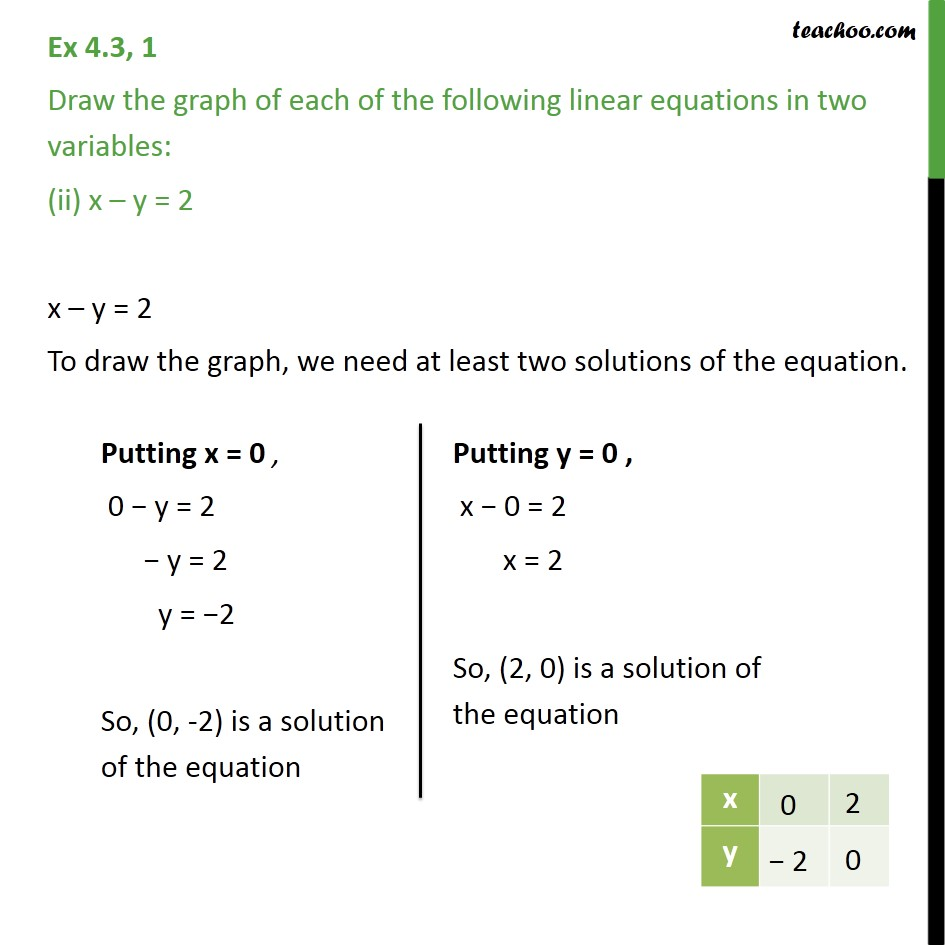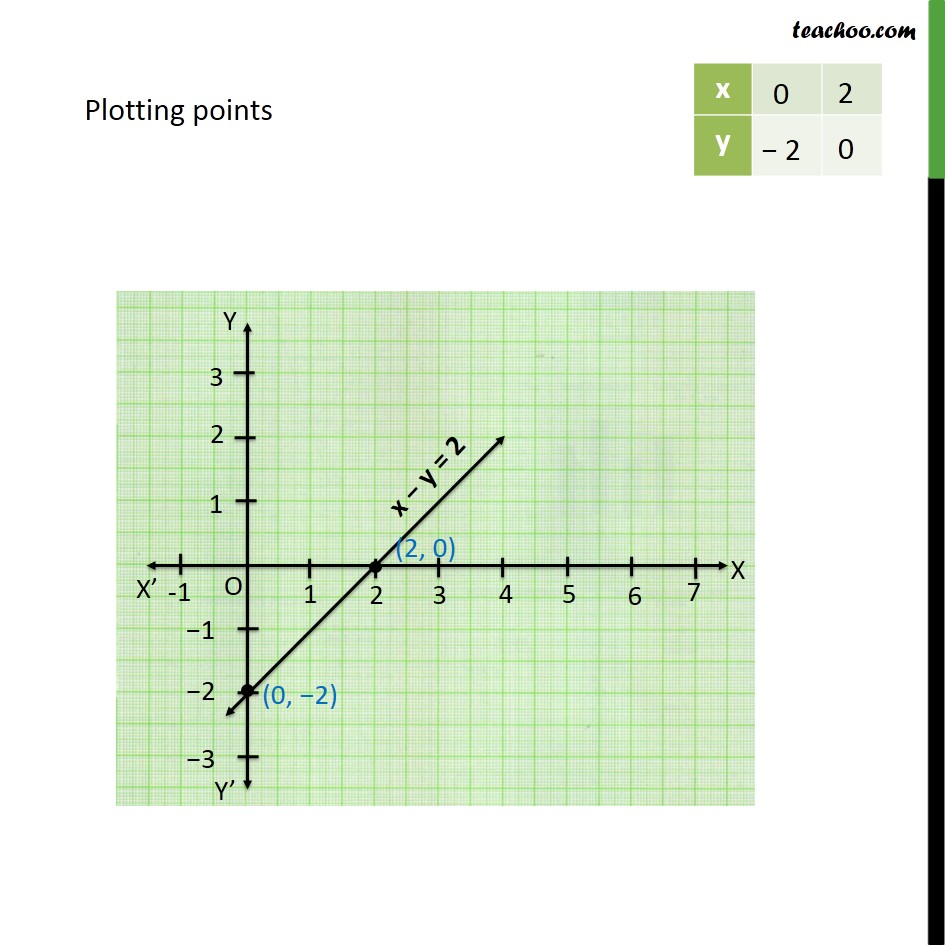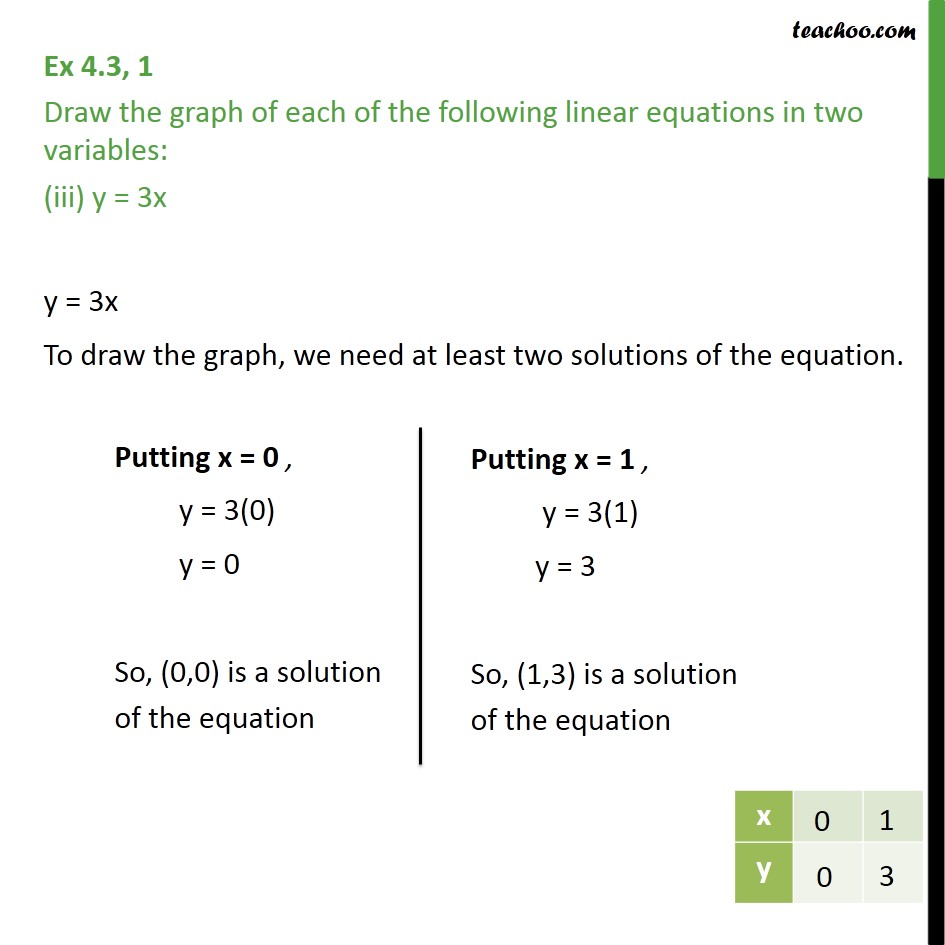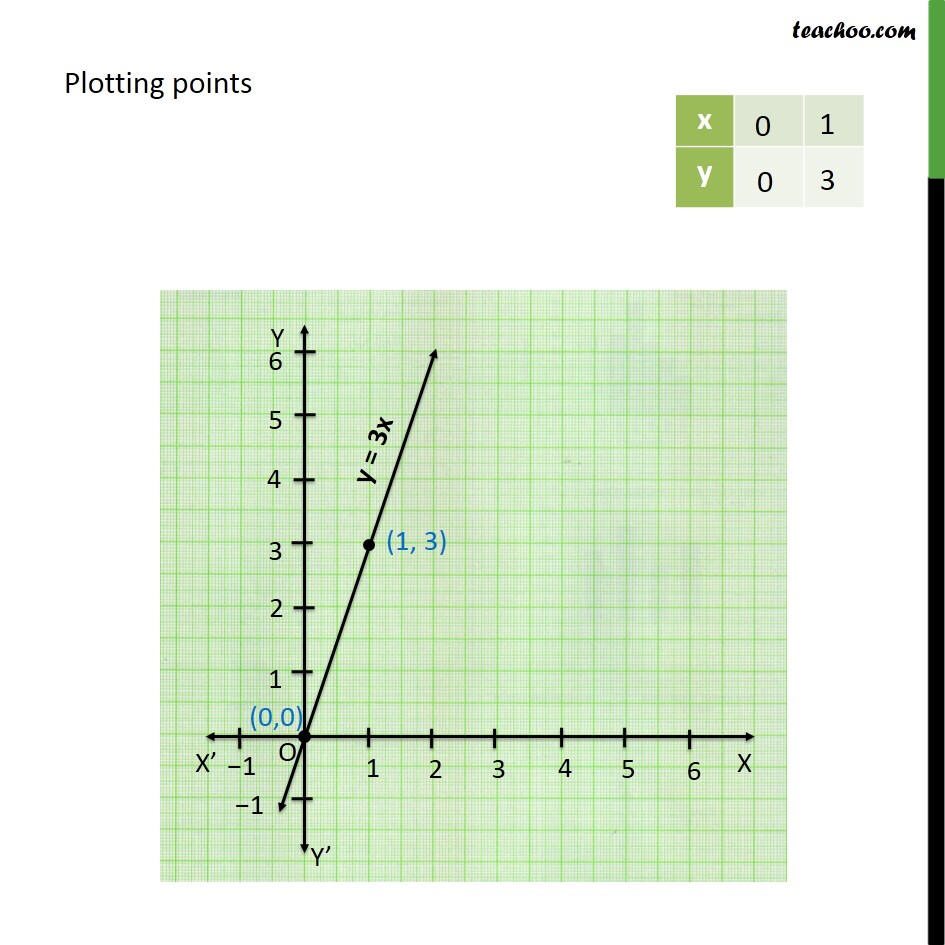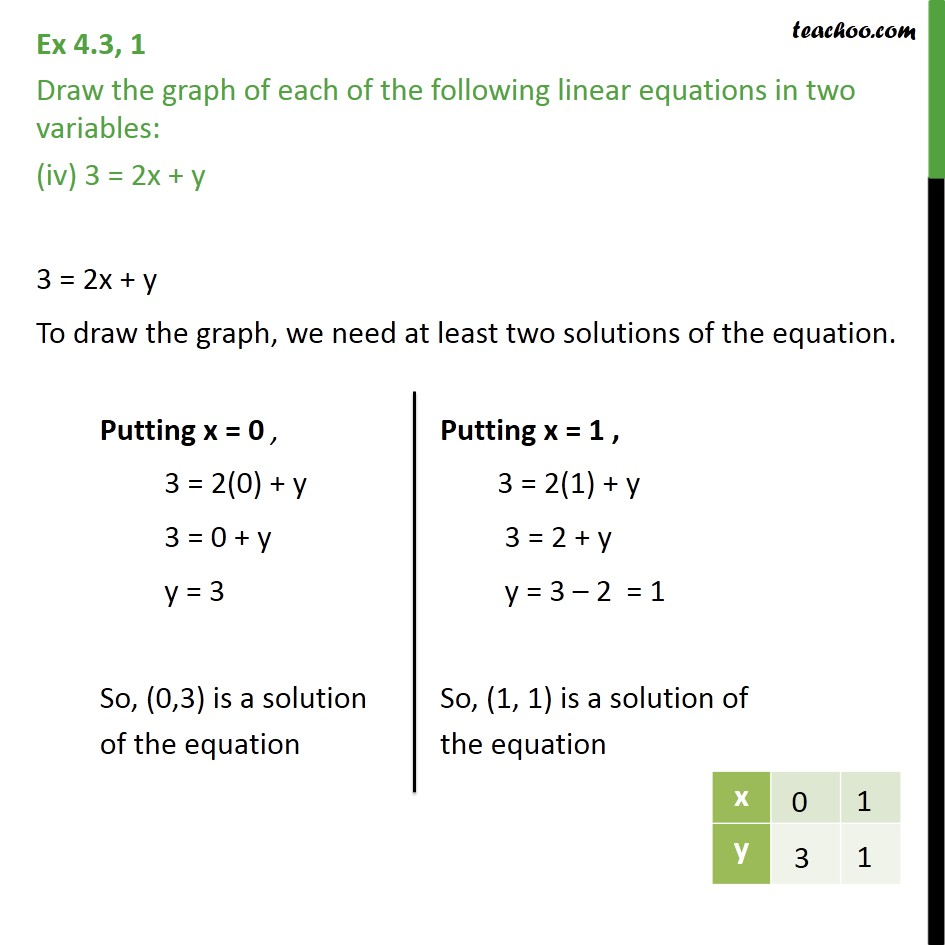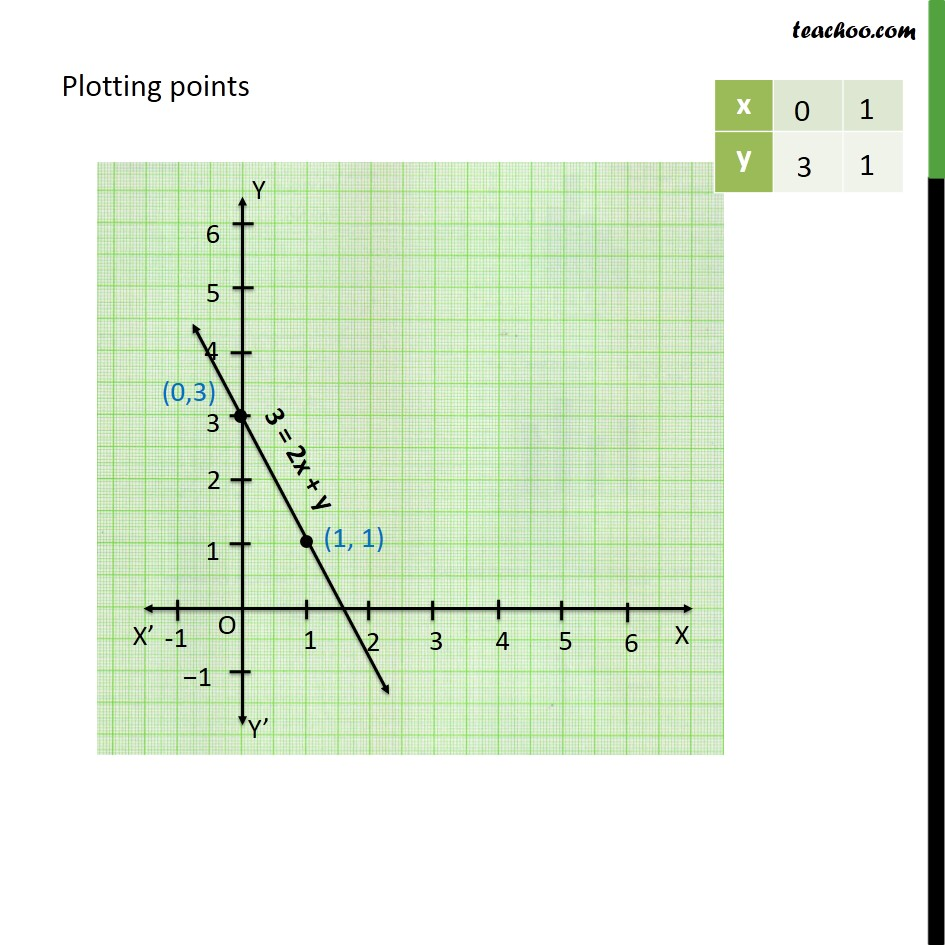1. Chapter 4 Class 9 Linear Equations in Two Variables
2. Serial order wise
3. Ex 4.3

Transcript

Ex 4.3, 1 Draw the graph of each of the following linear equations in two variables: x + y = 4 x + y = 4 To draw the graph, we need at least two solutions of the equation. Plotting points Ex 4.3, 1 Draw the graph of each of the following linear equations in two variables: (ii) x – y = 2 x – y = 2 To draw the graph, we need at least two solutions of the equation. Ex 4.3, 1 Draw the graph of each of the following linear equations in two variables: (iii) y = 3x y = 3x To draw the graph, we need at least two solutions of the equation. Plotting points Ex 4.3, 1 Draw the graph of each of the following linear equations in two variables: (iv) 3 = 2x + y 3 = 2x + y To draw the graph, we need at least two solutions of the equation. Plotting points

Ex 4.3